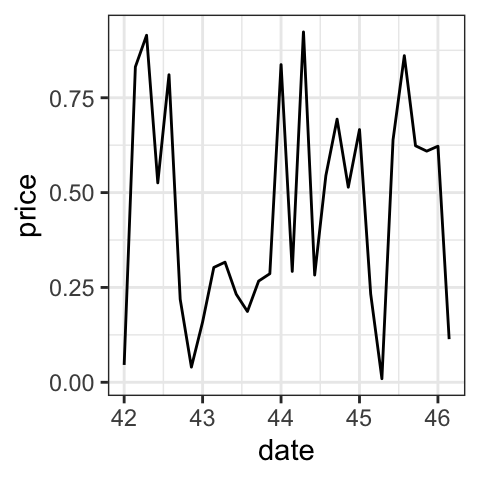# GGPlot Date Axis Customization#### GGPlot Date Axis Customization

This article describes how to format ggplot date axis using the R functions scale_x_date() and scale_y_date().

In this R graphics tutorial, you’ll learn how to:

• Change date axis labels using different combinations of days, weeks, months, year
• Modify date axis limits.

Contents:

## Key ggplot2 R functions

• scale_x_date(date_labels, limits) and scale_y_date(date_labels, limits): Format date axes
• scale_x_datetime(date-labels, limits) and scale_y_datetime(date_labels, limits): Format a datetime axis

## Time series data

Create some time series data sets:

set.seed(1234)
last_month <- Sys.Date() - 0:29
df <- data.frame(
date = last_month,
price = runif(30)
)
head(df)
##         date price
## 1 2018-11-13 0.114
## 2 2018-11-12 0.622
## 3 2018-11-11 0.609
## 4 2018-11-10 0.623
## 5 2018-11-09 0.861
## 6 2018-11-08 0.640

## Create a simple ggplot with date axis

library(ggplot2)
p <- ggplot(data=df, aes(x = date, y = price)) +
geom_line()
p## Format date axis labels: scale_x_date

To format date axis labels, you can use different combinations of days, weeks, months and years:

• Weekday name: use %a and %A for abbreviated and full weekday name, respectively
• Month name: use %b and %B for abbreviated and full month name, respectively
• %d: day of the month as decimal number
• %U: week of the year as decimal number (00–53)
• %Y: Year with century.
• See more options in the documentation of the function ?strptime
# Format : month/day
p + scale_x_date(date_labels = "%b/%d")

# Format : Week
p + scale_x_date(date_labels = "%U")

# Months only
p + scale_x_date(date_labels = "%B")+
theme(axis.text.x = element_text(angle=45, hjust = 1))## Set date axis limits

Use the economics time series data sets [in ggplot2]:

data("economics")
# Base plot with date axis
p <- ggplot(data = economics, aes(x = date, y = psavert)) +
geom_line(color = "steelblue")
p

# Set axis limits c(min, max)
min <- as.Date("2002-1-1")
max <- NA
p + scale_x_date(limits = c(min, max))## Conclusion

To change the format of data axis labels, first read the help page of the R base function strptime() to see the available date format.

Then, use the following example of R code:

p + scale_x_date(date_labels = "%b/%d")`